• The processor can usually address a memory space that is much larger than the memory space covered by an individual memory chip.

• In order to splice a memory device into the address space of the processor, decoding is necessary.

• For example, the 8088 issues 20-bit addresses for a total of 1MB of memory address space.

• However, the BIOS on a 2716 EPROM has only 2KB of memory and 11 address pins.

• A decoder can be used to decode the additional 9 address pins and allow the EPROM to be placed in any 2KB section of the 1MB address space.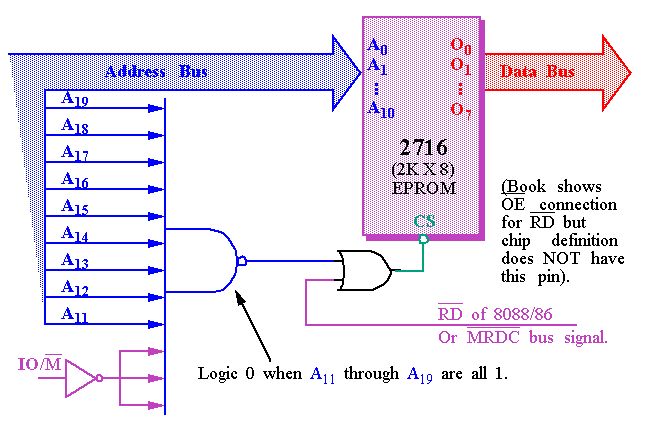• To determine the address range that a device is mapped into: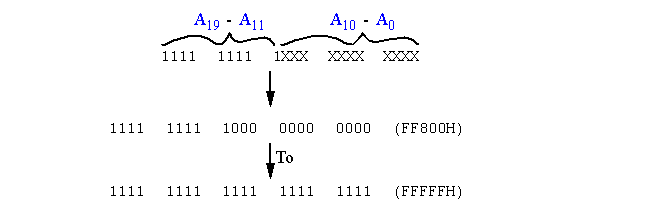• This 2KB memory segment maps into the reset location of the 8086/8088 (FFFF0H).

• NAND gate decoders are not often used.
• Rather the 3-to-8 Line Decoder (74LS138) is more common.

• The 3-to-8 Line Decoder (74LS138)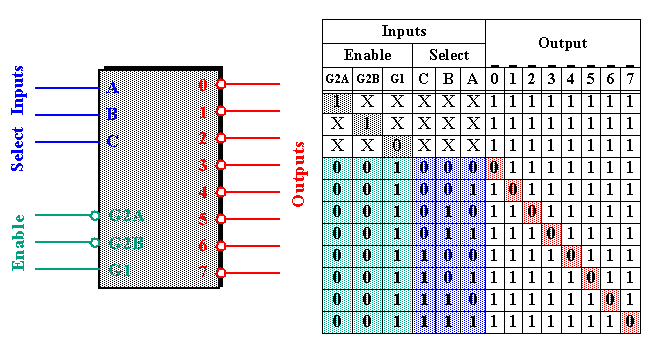• Note that all three Enables (G2A, G2B, and G1) must be active, e.g. low, low and high, respectively.
• Each output of the decoder can be attached to an 2764 EPROM ( 8K X 8 ).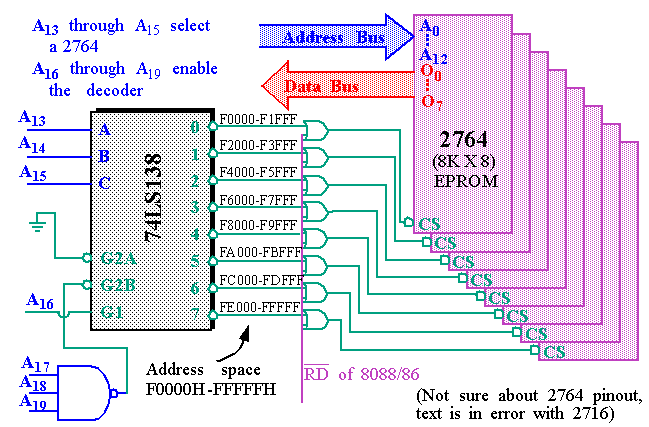• The EPROMs cover a 64KB section of memory.

• Yet a third possibility is a PLD (Programmable Logic Device).
• PLDs come in three varieties:
• PLA (Programmable Logic Array)
• PAL (Programmable Array Logic)
• GAL (Gated Array Logic)

• PLDs have been around since the mid-1970s but have only recently appeared in memory systems (PALs have replaced PROM address decoders).

• PALs and PLAs are fuse-programmed (like the PROM).
• Some are erasable (like the EPROM).

• A PAL example (16L8) is shown in the text and is commonly used to decode the memory address, particularly for 32-bit addresses generated by the 80386DX and above.

• AMD 16L8 PAL decoder.
• It has 10 fixed inputs (Pins 1-9, 11), two fixed outputs (Pins 12 and 19) and 6 pins that can be either (Pins 13-18).• AND/NOR device with logic expressions (outputs) with up to 16 ANDed inputs and 7 ORed product terms.

## 8088 and 80188 (8-bit) Memory Interface

• The memory systems "sees" the 8088 as a device with:
• 20 address connections (A19 to A0).
• 3 control signals, IO/M, RD, and WR.

• We'll look at interfacing the 8088 with:
• 32K of EPROM (at addresses F8000H through FFFFFH).
• 512K of SRAM (at addresses 00000H through 7FFFFH).

• The EPROM interface uses a 74LS138 (3-to-8 line decoder) plus 8 2732 ( 4K X 8 ) EPROMs.

• The EPROM will also require the generation of a wait state.
• The EPROM has an access time of 450ns .
• The 74LS138 requires 12ns to decode.
• The 8088 runs at 5MHz and only allows 460ns for memory to access data.

## 8088 and 80188 (8-bit) EPROM Memory Interface• The 8088 cold starts execution at FFFF0H . JMP to F8000H occurs here.

## 8088 and 80188 (8-bit) RAM Memory Interface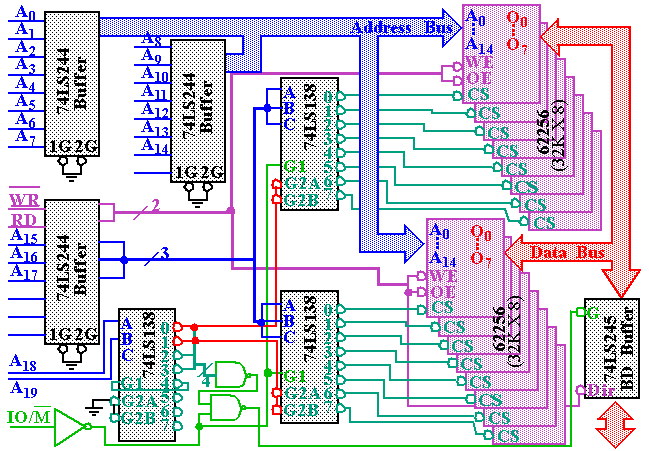## 8088 and 80188 (8-bit) RAM Memory Interface

• The 16 62256s on the previous slide are actually SRAMs.
• Access times are on order of 10ns .

• Flash memory can also be interfaced to the 8088 (see text).
• However, the write time ( 400ms !) is too slow to be used as RAM (as shown in the text).

• Parity Checking:
• Parity checking is used to detect single bit errors in the memory.

• The current trend is away from parity checking.

• Parity checking adds 1 bit for every 8 data bits.
• For EVEN parity, the 9th bit is set to yield an even number of 1's in all 9 bits.
• For ODD parity, the 9th bit is set to make this number odd.

• For 72-pin SIMMs, the number of data bits is 32 + 4 = 36 ( 4 parity bits).

## Parity for Memory Error Detection

• 74AS280 Parity Generator/Checker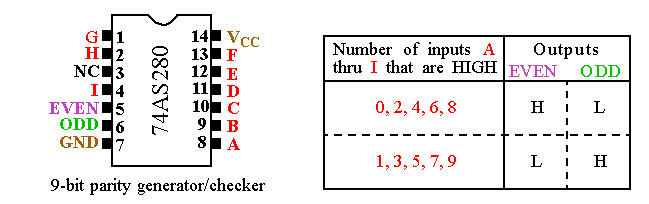• This circuit generates EVEN or ODD parity for the 9-bit number placed on its inputs.
• Typically, for generation, the 9th input bit is set to 0.

• This circuit also checks EVEN or ODD parity for the 9-bit number.
• In this case, the 9th input bit is connected to the 9th bit of memory.
• For example, if the original byte has an even # of 1's (with 9th bit at GND), the parity bit is set to 1 (from the EVEN output).
• If the EVEN output goes high during the check, then an error occurred.

## Parity for Memory Error Detection## Error Detection

• This parity scheme can only detect a single bit error.
• Block-Check Character ( BCC ) or Checksum.
• Can detect multiple bit errors.
• This is simply the two's complement sum (the negative of the sum) of the sequence of bytes.
• No error occurred if adding the data values and the checksum produces a 0.
• For example: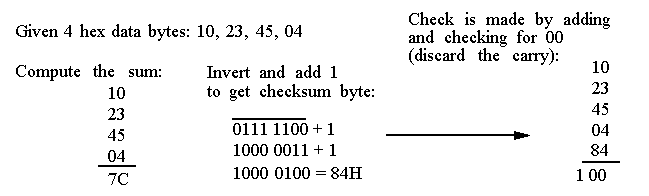• This is not fool proof.
• If 45 changes to 44 AND 04 changes to 05, the error is missed.

## Error Detection

• Cyclic Redundancy Check ( CRC ).
• Commonly used to check data transfers in hardware such as harddrives.
• Treats data as a stream of serial data n-bits long.
• The bits are treated as coefficients of a characteristic polynomial , M(X) of the form: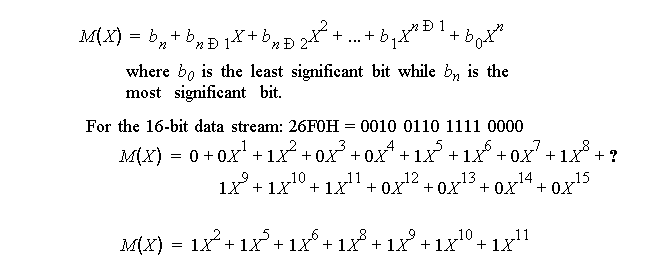## Error Detection

• Cyclic Redundancy Check ( CRC ) (cont.)
• The CRC is found by applying the following equation.• G(X) is the called the generator polynomial and has special properties.
• A commonly used polynomial is: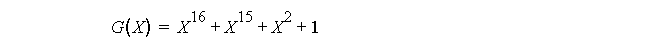• The remainder R(X) is appended to the data block.
• When the CRC and R(X) is computed by the receiver, R(X) should be zero.
• Since G(X) is of power 16, the remainder, R(X) , cannot be of order higher than 15.
• Therefore, no more than 2 bytes are needed independent of the data block size.

## Error Detection

• Cyclic Redundancy Check ( CRC )(cont.)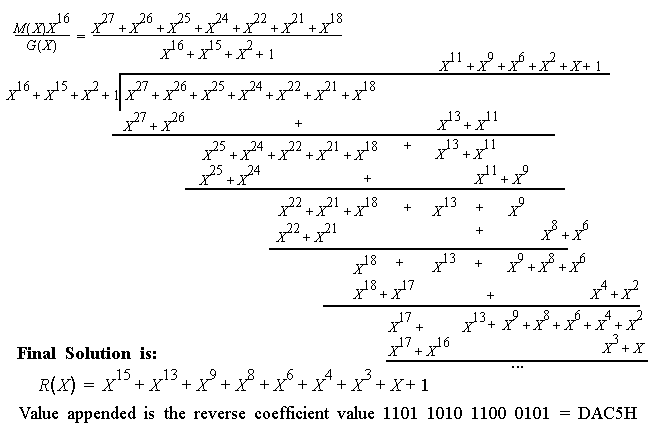## Error Correction

• Parity , BCC and CRC are only mechanisms for error detection.
• The system is halted if an error is found in memory.

• Error correction is starting to show up in new systems.
• SDRAM has ECC (Error Correction Code).

• Correction will allow the system can continue operating.
• If two errors occur, they can be detected but not corrected .
• Error correction will of course cost more in terms of extra bits.

• Error correction is based on Hamming Codes .
• There is lots of theory here but our focus will be on implementation.
• The objective is to correct any single bit errors in an 8-bit data byte.• In other words, we need 4 parity bits to correct single bit errors.
• Note that the parity bits are at bit positions that are powers of 2 .

## Error Correction

• Hamming Codes (cont).
• P1 is generated by computing the parity of X 3 , X 5 , X 7 , X 9 , X 11 , X 13 , X 15 .
• These numbers have a 1 in bit position 1 of the subscript in binary.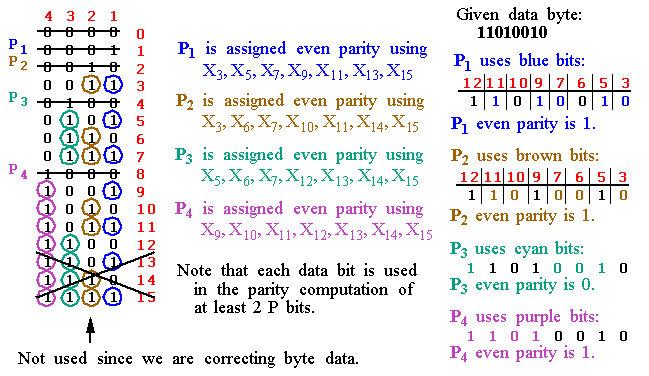## Error Correction

• Hamming Codes (cont).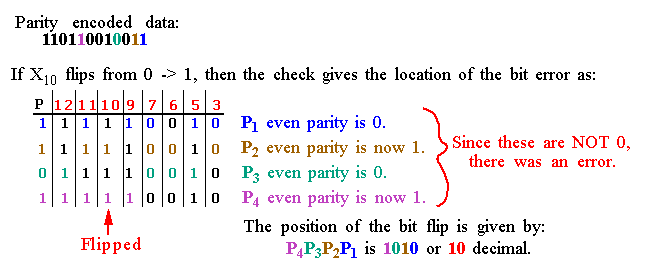## Parity for Memory Error Correction

• The 74LS636 corrects errors by storing 5 parity bits with each byte of data.
• The pinout consists of:
• 8 data I/O pins
• 5 check bit I/O pins
• 2 control pins
• 2 error outputs
• Single error flag ( SEF )
• Double error flag ( DEF ).• See the text for an example of its use in a circuit.